# Surface impedance condition (3D)

## Introduction

For a 3D application where the skin effect is pronounced, it is recommended to use the surface impedance formulation.

The surface impedance condition is a particular boundary condition, which takes into account the conducting volume regions solely by their boundaries, in the case when the skin effect is pronounced.

The surface impedance condition imposes a relation between the electric field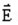and the magnetic fieldat the surface of the conducting region.

## Hypothesis

A hypothesis is created of a null electric field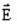throughout the conductor, except for the surface of the conducting region, where a surface current densityexists.

## Definition

The surface impedance (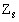) relates the tangential component of the magnetic field with the tangential component of the electric field at the surface of the conducting region, using the following relation: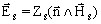, withwhere:

•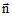is the unity vector normal to surface and exiting from the conducting region
• the index “s” signifies points placed on the surface of the conducting region, where the electric and magnetic fields are tangential.## Validity and limitations

There exist the following limitations for using the surface impedance condition:

• limitation of topological order: connectivity problem
• limitation of geometric order: The value of the skin depth δ must be small with respect to the conductor dimensions:
• δ <<L, where L is the significant length or width of the conducting region
• δ <<r, where r is the significant curvature radius of the conducting region

This limitation can be written as: δ <L/5 and δ < r/5

• limitation of physical order:

The field must be mainly tangent to the surface of the conducting region (negligible normal components of the field at the surface).

This limitation can be written: δ /L>10(μ0/μ) and μ >10μ0

## Complete information

Detailed information about the surface impedance condition is included in the “Time-harmonic magnetodynamics” chapter of volume 5 (see § 2.3.4).

## Interest

This formulation is a very economical one and it provides reliable results.

## Description of the solid conductor region

A conducting region with a pronounced skin effect is defined using: Region of solid conductor type described by surface impedance

A conducting region described by surface impedance is the combination of:

• an inactive volume region
• the boundary of the region on which the impedance surface condition is applied

The magnetic field is tangent to the boundary.The state variable(s) are not computed on the internal nodes of the volume region (inactive). Therefore, it is not necessary to mesh this region.

## Boundary conditions

Similarly, it is possible to apply the surface impedance condition on the computation domain boundary (of thin region type).